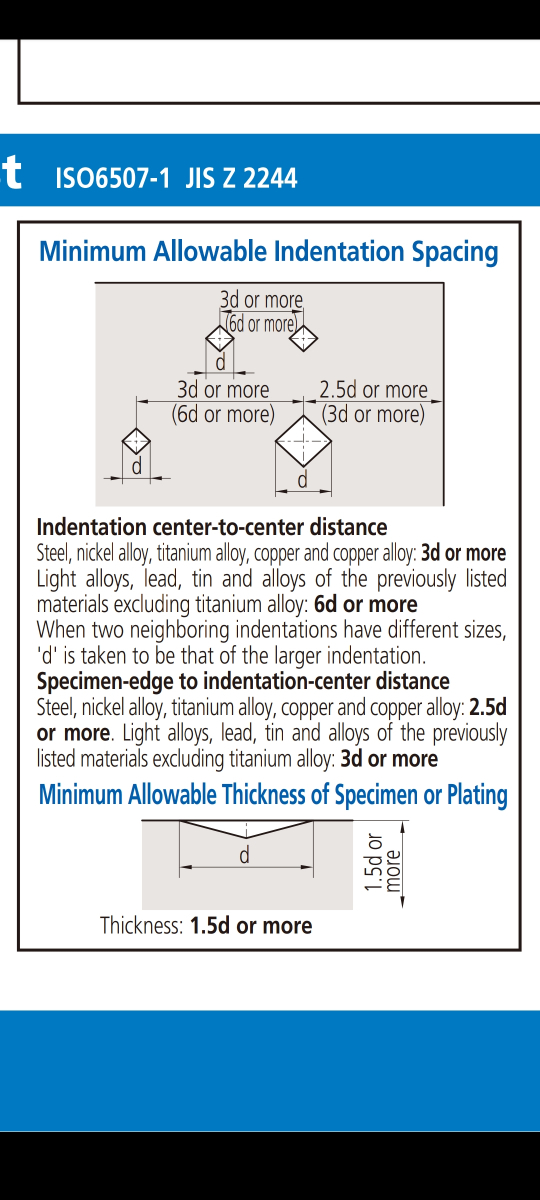# Vickers hardness: Distance between indentations

2 posts / 0 new
salveamigos123
Vickers hardness: Distance between indentations

Hello,
I'm writing here because I don't know how to solve this question that appeared on an exam (undergraduate, mechanical engineering).

The Vickers hardness test was done on a steel plate twice, once with a smaller force and once with a larger force. The first average length of the diagonal is d=0.181 mm. The second indent (larger force was used this time) left the average length of the diagonal d=0.572 mm. What is the minimum distance between indentations?

The equation we are using is B>3*d , where B is the distance between indentations, d is the average length of the diagonal.

Do I just take the average value for d of 0.181 mm and 0.572 mm = 0.3765 mm? Then B>3*0.3765= 1.13 mm?

I would be grateful if someone could help me with this.

Engr JaydeeHi @salveamigos123!

I think this reply is very late already, but I will post here for the sake of future mechanical engineering students encountering the same problem.

I found the above picture on a certain website. From this, the answer to your question is that $d$ is taken as the larger of the two diagonals, which is $d=0.572$ mm. Therefore, $$B>3d=3(0.572)=1.716\text{ mm}$$

• Mathematics inside the configured delimiters is rendered by MathJax. The default math delimiters are $$...$$ and $...$ for displayed mathematics, and $...$ and $...$ for in-line mathematics.